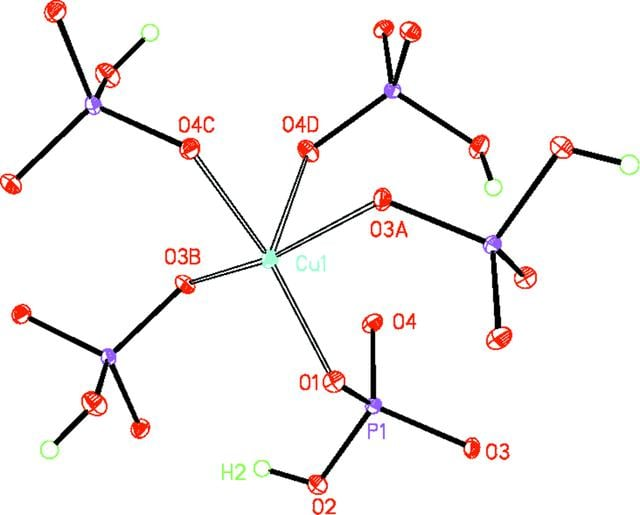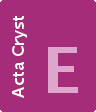disable zoom     view article Figure 1 The mol­ecular structure of 1, showing 50% probability displacement ellipsoides and the numbering scheme for the complete coordination polyhedron about Cu1 (Symmetry codes: (A) z,x − 1,y − 1; (B) −z + 2,-x + 2,-y + 1; (C) −x + 2,-y + 1,-z + 1 and (D) y,z,x − 1.)CRYSTALLOGRAPHICCOMMUNICATIONS
ISSN: 2056-9890
Follow Acta Cryst. E